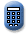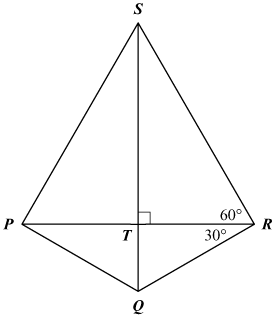Massachusetts Comprehensive Assessment SystemReporting Category: Geometry
Standard: 10.G.6 - Use the properties of special triangles (e.g., isosceles, equilateral, 30°-60°-90°, 45°-45°-90°) to solve problems.
Standard: CCSS.Math.Content.HSG-SRT.C.6 - Understand that by similarity, side ratios in right triangles are properties of the angles in the triangle, leading to definitions of trigonometric ratios for acute angles.

Kite PQRS has diagonals that intersect at point T. The kite and some of its angle measures are shown in the diagram below.The length of $\overline{RS}$ is 20 centimeters.

1. What is the length, in centimeters, of $\overline{PS}$? Show or explain how you got your answer.
2. What is the length, to the nearest tenth of a centimeter, of $\overline{ST}$? Show or explain how you got your answer.
3. What is the length, to the nearest tenth of a centimeter, of $\overline{TQ}$? Show or explain how you got your answer.
4. What is the perimeter, to the nearest tenth of a centimeter, of kite PQRS? Show or explain how you got your answer.

## Scoring Guide and Sample Student Work

Select a score point in the table below to view the sample student response.

ScoreDescription
4 The student response demonstrates an exemplary understanding of the Geometry concepts involved in understanding that by similarity, side ratios in right triangles are properties of the angles in the triangle, leading to definitions of trigonometric ratios for acute angles. The student finds the perimeter of a kite that is composed of special right angles.
4
3 The student response demonstrates a good understanding of the Geometry concepts involved in understanding that by similarity, side ratios in right triangles are properties of the angles in the triangle, leading to definitions of trigonometric ratios for acute angles. Although there is significant evidence that the student was able to recognize and apply the concepts involved, some aspect of the response is flawed. As a result the response merits 3 points.
2 The student response demonstrates a fair understanding of the Geometry concepts involved in understanding that by similarity, side ratios in right triangles are properties of the angles in the triangle, leading to definitions of trigonometric ratios for acute angles. While some aspects of the task are completed correctly, others are not. The mixed evidence provided by the student merits 2 points.
1 The student response demonstrates a minimal understanding of the Geometry concepts involved in understanding that by similarity, side ratios in right triangles are properties of the angles in the triangle, leading to definitions of trigonometric ratios for acute angles.
0 The student response contains insufficient evidence of an understanding of the Geometry concepts involved in understanding that by similarity, side ratios in right triangles are properties of the angles in the triangle, leading to definitions of trigonometric ratios for acute angles to merit any points.
Note: There are 2 sample student responses for Score Point 4.

Question 17: Number and Quantity
Question 20: Algebra and Functions
Question 21: Algebra and Functions
Question 36: Geometry
Question 41: Statistics and Probability
Question 42: Geometry

Last Updated: April 8, 2022